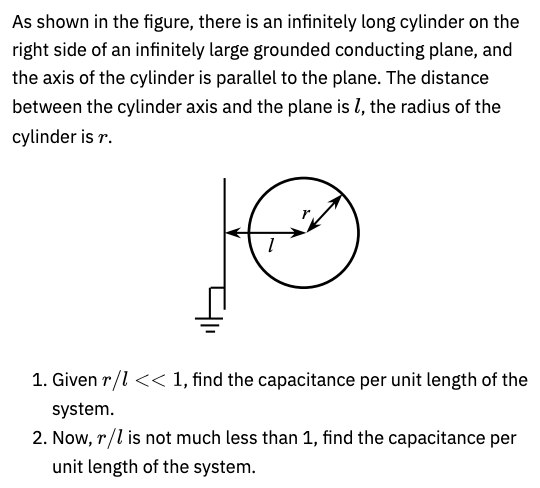# Capacitance between infinite plane and cylinderPlease provide a solution for this problem. Thank you!

If the conducting plane is grounded then its potential \varphi=0. You should use the method of images and imagine that there’s another cylinder on the other side of the grounded plane. Then use the formula \varphi_2-\varphi_1=-\int\limits_{1}^{2}\vec E(\vec r')\vec {dr'} to find the potential difference between the cylinder and its image. You can assume that the capacitance per unit length is C=\frac{\lambda }{V}

5 лайков

Thanks!

Also there is a part B.1 from T1 of the APhO 2019 which is very similar to this problem.

2 лайка

Oh I see, let me have a look!

1 лайк